Courses

# Test: Aromatic Electrophilic Substitution

## 25 Questions MCQ Test Chemistry for JEE | Test: Aromatic Electrophilic Substitution

Description
This mock test of Test: Aromatic Electrophilic Substitution for JEE helps you for every JEE entrance exam. This contains 25 Multiple Choice Questions for JEE Test: Aromatic Electrophilic Substitution (mcq) to study with solutions a complete question bank. The solved questions answers in this Test: Aromatic Electrophilic Substitution quiz give you a good mix of easy questions and tough questions. JEE students definitely take this Test: Aromatic Electrophilic Substitution exercise for a better result in the exam. You can find other Test: Aromatic Electrophilic Substitution extra questions, long questions & short questions for JEE on EduRev as well by searching above.
QUESTION: 1

### Direction (Q. Nos. 1 - 10) This is section contains 10 multiple choice questions. Each question has four choices (a), (b), (c) and (d) out of which ONLY ONE option is correct. Q. The electrophile in sulphonation of benzene using fuming sulphuric acid is

Solution:

The correct answer is Option B.
Fuming sulphuric acid, H2S2O7, can be thought of as a solution of SO3 in sulphuric acid - and so is a much richer source of the SO3. Sulphur trioxide is an electrophile because it is a highly polar molecule with a fair amount of positive charge on the sulphur.

QUESTION: 2

### In nitration of benzene using cone. H2SO4 and cone. HNO3, the role of nitric acid is

Solution:

The correct answer is Option B.
Nitration is electrophilic substitution reaction; in its first step HNO3 takes protons from sulphuric acid and then forms −NO2+ so here HNO3 acts as base.

Mechanism of reaction as shown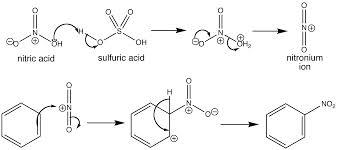QUESTION: 3

### The increasing order of reactivity of the following compounds towards electrophilic aromatic substitution reaction is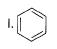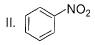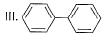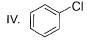Solution:
QUESTION: 4

What is the correct increasing order of reactivity of the following compounds towards electrophilic aromatic substitution reaction?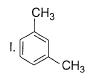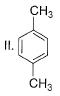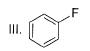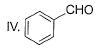Solution:

The correct answer is option B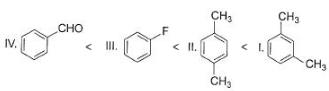QUESTION: 5

What is major product of the reaction below?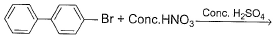Solution:

The correct answer is option D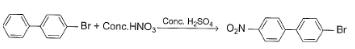QUESTION: 6

Predict major product of the following reaction.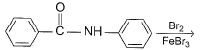Solution:
QUESTION: 7

Which of the following act as electrophile in halogenation?

Solution:

Halonium ion act as electrophile in halogenation. Nitronium ion is used in nitration. Sulphonium ion is used in sulphonation. Acylium ion is used in acylation.

QUESTION: 8

Identify the correct order of reactivity of following towards aromatic electrophilic substitution reaction.

I. Toluene
II. Benzene
III. Chlorobenzene
IV. Nitrobenzene

Solution:

The correct answer is Option A
Toluene is having one methyl group which is an electron-donating group causing a negative charge on the carbon atom of the ring so it is highly reactive towards electrophile which is already electron deficient.
Benzene has a delocalised set of electron clouds which attracts electrophile while chloro and nitro group are electronegative causing positive charge on carbon atoms so are not reactive towards electrophilic substitution.
Cl shows -I effect and −NO2 shows a strong electron-withdrawing effect. So least reactive
a)IV < III < II < I

QUESTION: 9

Predict the major product in the following reaction.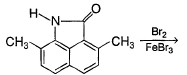[IIT JEE 2001]

Solution:
QUESTION: 10

What is true about sulphonation of C6H6?

Solution:
*Multiple options can be correct
QUESTION: 11

Direction (Q. Nos. 11 - 15) This section contains 5 multiple choice questions. Each question has four choices (a), (b), (c) and (d), out of which ONE or MORE THAN ONE are correct.

Q. What is/are true regarding following reaction?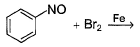Solution:
*Multiple options can be correct
QUESTION: 12

What is/are true about chlorination of toluene?

Solution:
*Multiple options can be correct
QUESTION: 13

Consider the following reaction,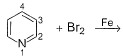The correct statement(s) is/are

Solution:
QUESTION: 14

Which among these is not a representative arene compound?

Solution:
*Multiple options can be correct
QUESTION: 15

The compound(s) that undergo slower electrophilic substitution than benzene is/are

Solution:
QUESTION: 16

Direction (Q. Nos. 16 - 21) This section contains 2 paragraphs, each describing theory, experiments, data, etc. Six questions related to the paragraphs have been given. Each question has only one correct answer among the four given options (a), (b), (c) and (d).

Passage I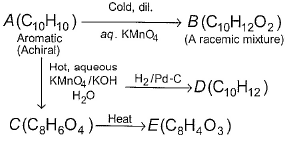Q. The most likely structure of A is

Solution:
QUESTION: 17

Passage IQ. What is correct about D?

Solution:
QUESTION: 18

Passage IQ. If A is treated with cone. HNO3 and cone. H2SO4 mixture, mononitration at phenyl ring takes place. What is the structure of major nitration product?

Solution:
QUESTION: 19

Passage II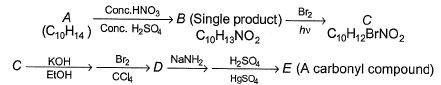Q. The most likely structure of A is

Solution:
QUESTION: 20

Passage IIQ. What is true about C?

Solution:
QUESTION: 21

Passage IIQ. What is the structure of E?

Solution:
*Answer can only contain numeric values
QUESTION: 22

Direction (Q. No. 22 - 25) This section contains 4 questions. When worked out will result in an integer from 0 to 9 (both inclusive).

Q. C6H4Br2(P) is a dibromo benzene and it is the less polar isomer of its two polar isomers. If P is treated with conc. HNO3 and conc -H2SO4 mixture, in principle, how many mono nitration products are expected?

Solution:
*Answer can only contain numeric values
QUESTION: 23

How many isomers of C7H8O exists that has a phenyl ring?

Solution:

C7H8 is toluene: that’s the only benzenoid isomer possible. To get C7H8O, you must mentally “insert an oxygen atom” somewhere.
There are 5 ways you can mentally insert an oxygen atom into a C-C or C-H bond of toluene.
So the isomers of C7H8O are:
2-methylphenol = o-cresol
3-methylphenol = m-cresol
4-methylphenol = p-cresol
benzyl alcohol = phenylmethanol = (hydroxymethyl)benzene
methoxybenzene = anisole = methyl phenyl ether

*Answer can only contain numeric values
QUESTION: 24

If the following compound is treated with Br2-Fe, how many mono bromination products are formed in principle?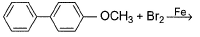Solution: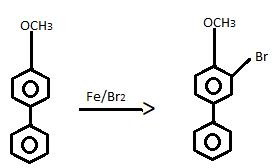*Answer can only contain numeric values
QUESTION: 25

How many of the following have an activated aromatic ring for electrophilic substitution reaction?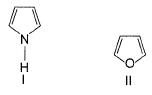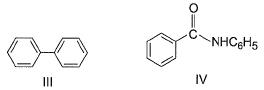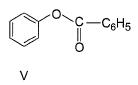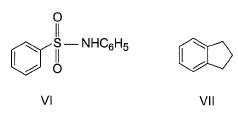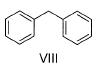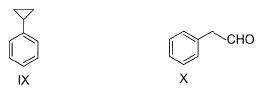Solution: